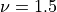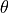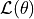# Kriging : draw the likelihood¶

## Abstract¶

In this short example we draw the loglikelihood as a function of the scale parameter of a covariance kriging model.

import openturns as ot
import openturns.viewer as otv


We define the exact model with a SymbolicFunction :

f = ot.SymbolicFunction(["x"], ["x*sin(x)"])


We use the following input and output training samples :

inputSample = ot.Sample([[1.0], [3.0], [5.0], [6.0], [7.0], [8.0]])
outputSample = f(inputSample)


We choose a constant basis for the trend of the metamodel :

basis = ot.ConstantBasisFactory().build()
covarianceModel = ot.SquaredExponential(1)


For the covariance model, we use a Matern model with:

covarianceModel = ot.MaternModel([1.0], 1.5)


We are now ready to build the kriging algorithm, run it and store the result :

algo = ot.KrigingAlgorithm(inputSample, outputSample, covarianceModel, basis)
algo.run()
result = algo.getResult()


We can retrieve the covariance model from the result object and then access the scale of the model :

theta = result.getCovarianceModel().getScale()
print("Scale of the covariance model : %.3e" % theta)

Scale of the covariance model : 1.978e+00


This hyperparameter is calibrated thanks to a maximization of the log-likelihood. We get this log-likehood as a function of:

ot.ResourceMap.SetAsBool(
"GeneralLinearModelAlgorithm-UseAnalyticalAmplitudeEstimate", True
)
reducedLogLikelihoodFunction = algo.getReducedLogLikelihoodFunction()


We draw the reduced loglikelihoodas a function of the parameter.

graph = reducedLogLikelihoodFunction.draw(0.1, 10.0, 100)
graph.setXTitle(r"$\theta$")
graph.setYTitle(r"$\mathcal{L}(\theta)$")
graph.setTitle(r"Log-likelihood as a function of $\theta$")


We represent the estimated parameter as a point on the loglikelihood curve :

L_theta = reducedLogLikelihoodFunction(theta)
cloud = ot.Cloud(theta, L_theta)
cloud.setColor("red")
cloud.setPointStyle("fsquare")
graph.setLegends([r"Matern $\nu = 1.5$", r"$\theta$ estimate"])


We verify on the previous graph that the estimate ofmaximizes the loglikelihood.

Display figures

view = otv.View(graph)
otv.View.ShowAll()Reset default settings

ot.ResourceMap.Reload()